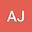loading page

Numerical Approximate Solution to Flow over a Flat Plate ( the Balusis Problem) By Perturbation Iteration- Algorithm
•## Abstract

This paper applied perturbation iteration- algorithm (PI-A) to solve the problem of the incompressible two-dimensional laminar boundary layer flow over a flat plat as wall as called the Blasius problem (BP). BP is governed by Navier- Stokes equation (NSE) and continuity equation which were transformed into ordinary differential equation using similarity transforms. The results presented are tabulated for similarity stream function and can be seen highly of accuracy through comparable with that obtained by Ganji et al. who studied BP using Homotopy perturbation technique (HPT) , Research results for the same problem using the variationally This paper applied perturbation iteration- algorithm (PI-A) to solve the problem of the incompressible two-dimensional laminar boundary layer flow over a flat plat as wall as called the Blasius problem (BP). BP is governed by Navier- Stokes equation (NSE) and continuity equation which were transformed into ordinary differential equation using similarity transforms. The results presented are tabulated for similarity stream function and can be seen highly of accuracy through comparable with that obtained by Ganja et al. who studied BP using Homotopy perturbation technique (HPT) , Research results for the same problem using the variational iteration technique (VIT) before Aiyesimi and Niyi and results numerical by Blasius. Finally, The method that is efficient and widely applicable for solving ODE.

#### Peer review status:UNDER REVIEW

08 Feb 2021Submitted to Mathematical Methods in the Applied Sciences
10 Feb 2021Assigned to Editor
10 Feb 2021Submission Checks Completed
13 Feb 2021Reviewer(s) Assigned